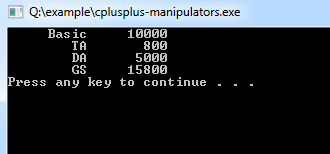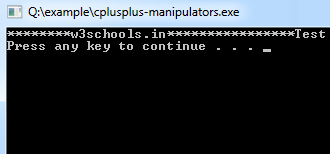# C++ Manipulators

Manipulators are operators used in C++ for formatting output. The data is manipulated by the programmer's choice of display.

Some of the more commonly used manipulators are given below:

## endl Manipulator

endl is the line feed operator in C++. It acts as a stream manipulator whose purpose is to feed the whole line and then point the cursor to the beginning of the next line. We can use \n (\n is an escape sequence) instead of endl for the same purpose.

## setw Manipulator

This manipulator sets the minimum field width on output.

Syntax:
``setw(x)``

Example:

``````/*
* File: main.cpp
* Author: Gautam
* Created on October 16, 2011, 12:58 PM
*/#include <iostream>
#include <iomanip>
using namespace std;

int main() {

float basic, ta,da,gs;
basic=10000; ta=800; da=5000;
gs=basic+ta+da;
cout<<setw(10)<<"Basic"<<setw(10)<<basic<<endl
<<setw(10)<<"TA"<<setw(10)<<ta<<endl
<<setw(10)<<"DA"<<setw(10)<<da<<endl
<<setw(10)<<"GS"<<setw(10)<<gs<<endl;
return 0;
}
``````
Program Output:## setfill Manipulator

This is used by the setw manipulator. If a value does not entirely fill a field, then the character specified in the setfill argument of the manipulator is used for filling the fields.

Example:
``````/*
* File: main.cpp
* Author: Gautam
* Created on October 16, 2011, 12:58 PM
*/#include <iostream>
#include <iomanip>
using namespace std;

int main() {
cout << setw(20) << setfill('*') << "w3schools.in" << setw(20) << setfill('*')<<"Test"<< endl;
}``````
Program Output: